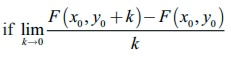Home | | Maths 12th Std | Summary

# Summary

maths : Differentials and Partial Derivatives: Summary

SUMMARY

ŌĆó Let f : (a, b) ŌåÆ ŌäØ be a differentiable function and x0 Ōłł (a, b) then linear approximation L of f at x0 is given by

L(x) = f (x0) + f  ŌĆ▓ (x0) (x - x0ŌłĆ x Ōłł (a,b)

ŌĆó Absolute error = Actual value ŌĆō Approximate value

Relative error = Absolute error / Actual value

Percentage error = Relative error ├Ś 100

(or)

Acutal value / Absolute error ├Ś 100

ŌĆó Let f : ( a , b)ŌåÆ ŌäØ be a differentiable function. For x Ōłł ( a,b) and ╬öx the increment given to x, the differential of f is defined by df = f ŌĆ▓( x ) ╬öx .

ŌĆó All the rules for limits (limit theorems) for functions of one variable also hold true for

functions of several variables.

ŌĆó Let A = {( x , y ) | a < x < b, c < y < d }ŌŖé ŌäØ2 , F : A ŌåÆ ŌäØ and ( x0 , y0 ) Ōłł A .

ŌĆó (i) F has a partial derivative with respect to x at ( x0 , y0 )Ōłł Aexists and it is denoted byF has a partial derivative with respect to y at ( x0 , y0 )Ōłłexists and limit value is defined byŌĆó ClariantŌĆÖs Theorem: Suppose that A = {( x , y ) a < x < b, c < y < d}ŌŖé ŌäØ2 , F : A ŌåÆ ŌäØ . If fxy and f yx exist in A and are continuous in A, then f xy = fyx in A.

ŌĆó Let A = {( x , y ) | a < x < b, c < y < d} ŌŖé ŌäØ2. A function U : A ŌåÆ ŌäØ is said to be harmonic in A if it satisfies Ōłé2u/Ōłéx2 + Ōłé2u/Ōłéy2 = 0, ŌłĆ( x , y)Ōłł A . This equation is called LaplaceŌĆÖs equation.

ŌĆó Let A = {( x , y ) | a < x < b, c < y < d}ŌŖé ŌäØ2 , F : A ŌåÆ ŌäØ and ( x0 , y0 )Ōłł A .

(i) The linear approximation of F at ( x0 , y0 )Ōłł A is defined to be(ii) The differential of F is defined to be dF = ŌłéF/Ōłéx dx + ŌłéF/Ōłéy dy where ╬öx = dx and ╬öy = dy .

ŌĆó Suppose w is a function of two variables x, y where x and y are functions of a single variable ŌĆśtŌĆÖ then  dw/dt = Ōłéw/Ōłéx Ōŗģ dxdt + Ōłéw/Ōłéy Ōŗģ dy/dt

ŌĆó Suppose w is a function of two variables x and y where x and y are functions of two variables s and t then,ŌĆó Suppose that A = {( x , y ) | a < x < b, c < y < d}ŌŖé ŌäØ2 , F : A ŌåÆ ŌäØ2 . If F is having continuous partial derivatives and homogeneous on A, with degree p, then x ŌłéF/Ōłéx + y ŌłéF/Ōłéy = pF.

Tags : Differentials and Partial Derivatives | Mathematics , 12th Maths : UNIT 8 : Differentials and Partial Derivatives
Study Material, Lecturing Notes, Assignment, Reference, Wiki description explanation, brief detail
12th Maths : UNIT 8 : Differentials and Partial Derivatives : Summary | Differentials and Partial Derivatives | Mathematics